# Multistage Rate Conversion

Multistage rate conversion is an approach that splits rate conversion into several stages. For example, instead of decimation by a factor of 18, decimate by factor of 3, followed by another decimation by 3, and and then by a factor of 2. Using multiple stages reduces the computational complexity of filtered rate conversion. Furthermore, if one already has the converter units for the different prime factors, they can be used as building blocks for higher rates. This example demonstrates multistage rate conversion designs.

### Single-Stage v.s. Multistage Conversion: Cost Analysis

Consider decimation system of rate M = 8. One can implement such a system in two ways:

• A single decimator of rate M = 8.

• A cascade of three half-rate decimators ( M = 2)

A multistage cascade of filtered decimators (or interpolators) has a reduced single-stage form. The filter in the reduced form is called the single-stage equivalent filter, which encapsualtes the filters of all the stages. Thus, any multistage cascade FIR decimator can be represented as a single-stage FIR decimator. For more details, see . However, while the two alternatives effectively perform the same decimation, they differ in their numerical complexites.

Evaluate the cost of implementing a multistage decimator using the cost function, and compare to the cost of implementing a single-stage decimator.

firDecim2_1 = dsp.FIRDecimator(2); firDecim2_2 = dsp.FIRDecimator(2); firDecim2_3 = dsp.FIRDecimator(2); firDecim2cascade = dsp.FilterCascade(firDecim2_1,firDecim2_2,firDecim2_3); cost2cascade = cost(firDecim2cascade) firDecim8 = dsp.FIRDecimator(8); cost8 = cost(firDecim8) 
cost2cascade = struct with fields: NumCoefficients: 75 NumStates: 138 MultiplicationsPerInputSample: 21.8750 AdditionsPerInputSample: 21 cost8 = struct with fields: NumCoefficients: 169 NumStates: 184 MultiplicationsPerInputSample: 21.1250 AdditionsPerInputSample: 21 

Cascading three decimators of rate M=2 consumes less memory (states and coefficients) compared to a single-stage decimator of M=8, making the multistage converter more memory efficient. The arithmetic load (operations per sample) of the single-stage and multistage implementation are equivalent. Note that the number of samples drops by half after each decimation stage. In conclusion, it is often better to split the decimation into multiple stages (given that the rate change factor is not a prime number, of course).

There is usually more than one way to factor a (non-prime) conversion rate, and even more degrees of freedom multistage design. The DSP System Toolbox (TM) offers several tools to simplify the design process. We will examine two of them in what follows.

### Using designMultistageDecimator and designMultistageInterpolator Functions

The designMultistageInterpolator and designMultistageDecimator functions automatically determine an optimal configuation, that includes determining the number of stages along with their arrangements, lowpass parameters, etc. The result is a filter cascade system object, which encapsualtes all the stages. To illustrate, let us design a decimator of rate M=12.

M = 12; fcDecMulti = designMultistageDecimator(M); disp(cascadeInfoSummary(fcDecMulti)) 
Multistage FIR Decimator, M=12 (48.0kHz to 4.0kHz) Equivalent lowpass cutoff: 4.0kHz, transition Width: 800.0Hz Number of stages: 3 Stage1: FIR Decimator, M = 2 (48.0kHz to 24.0kHz), FIR Length = 11 Stage2: FIR Decimator, M = 2 (24.0kHz to 12.0kHz), FIR Length = 15 Stage3: FIR Decimator, M = 3 (12.0kHz to 4.0kHz), FIR Length = 79 

This particular design has 3 stages (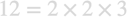), where the lowpass of the last stage is the longest.

Repeat the design with a single-stage.

fcDecSingle = designMultistageDecimator(M,'NumStages',1); disp(cascadeInfoSummary(fcDecSingle)) 
Multistage FIR Decimator, M=12 (48.0kHz to 4.0kHz) Equivalent lowpass cutoff: 4.0kHz, transition Width: 800.0Hz Number of stages: 1 Stage1: FIR Decimator, M = 12 (48.0kHz to 4.0kHz), FIR Length = 307 

Compare the cost of the two implementations. Obivously, the multistage approach is more efficient.

costMulti = cost(fcDecMulti) costSingle = cost(fcDecSingle) 
costMulti = struct with fields: NumCoefficients: 69 NumStates: 102 MultiplicationsPerInputSample: 10.1667 AdditionsPerInputSample: 9.3333 costSingle = struct with fields: NumCoefficients: 283 NumStates: 300 MultiplicationsPerInputSample: 23.5833 AdditionsPerInputSample: 23.5000 

Now, let us compare the combined frequency response of the decimation filters. While the filters of the two implementations differ in the stopband, the passband and transition band are nearly identical.

hfv = fvtool(fcDecMulti, fcDecSingle); legend(hfv,'Multistage Combined Response', 'Single-Stage Response');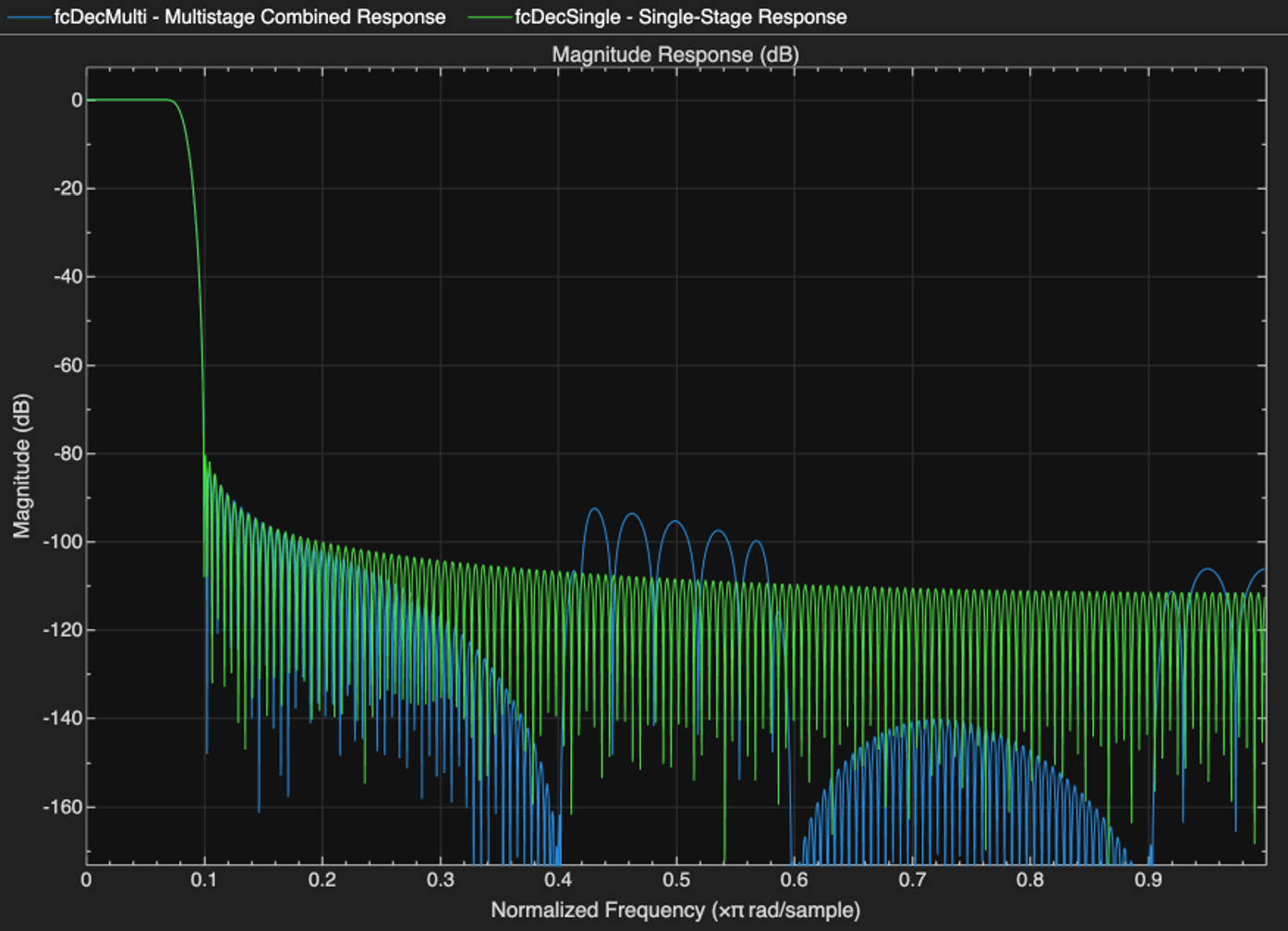The same methodology applies for designMultistageInterpolator. Create two interpolators (single-stage and multistage) and compare their outputs. Note that the outputs are nearly identical, except a slightly longer latency of the multistage interpolator.

n = (1:20)'; x = (abs(n-5)<=5).*(5-abs(n-5)); L = 12; fcIntrMulti = designMultistageInterpolator(L); fcIntrSingle = designMultistageInterpolator(L,'NumStages',1); xInterpSingle = fcIntrSingle(x); xInterpMulti = fcIntrMulti(x); release(fcIntrMulti); release(fcIntrSingle); subplot(3,1,1); stem(x); xlim([1,20]); title('Input Sequence'); subplot(3,1,2); stem(xInterpSingle); title('Single-Stage Interpolated') subplot(3,1,3); stem(xInterpMulti); title('Multistage Interpolated')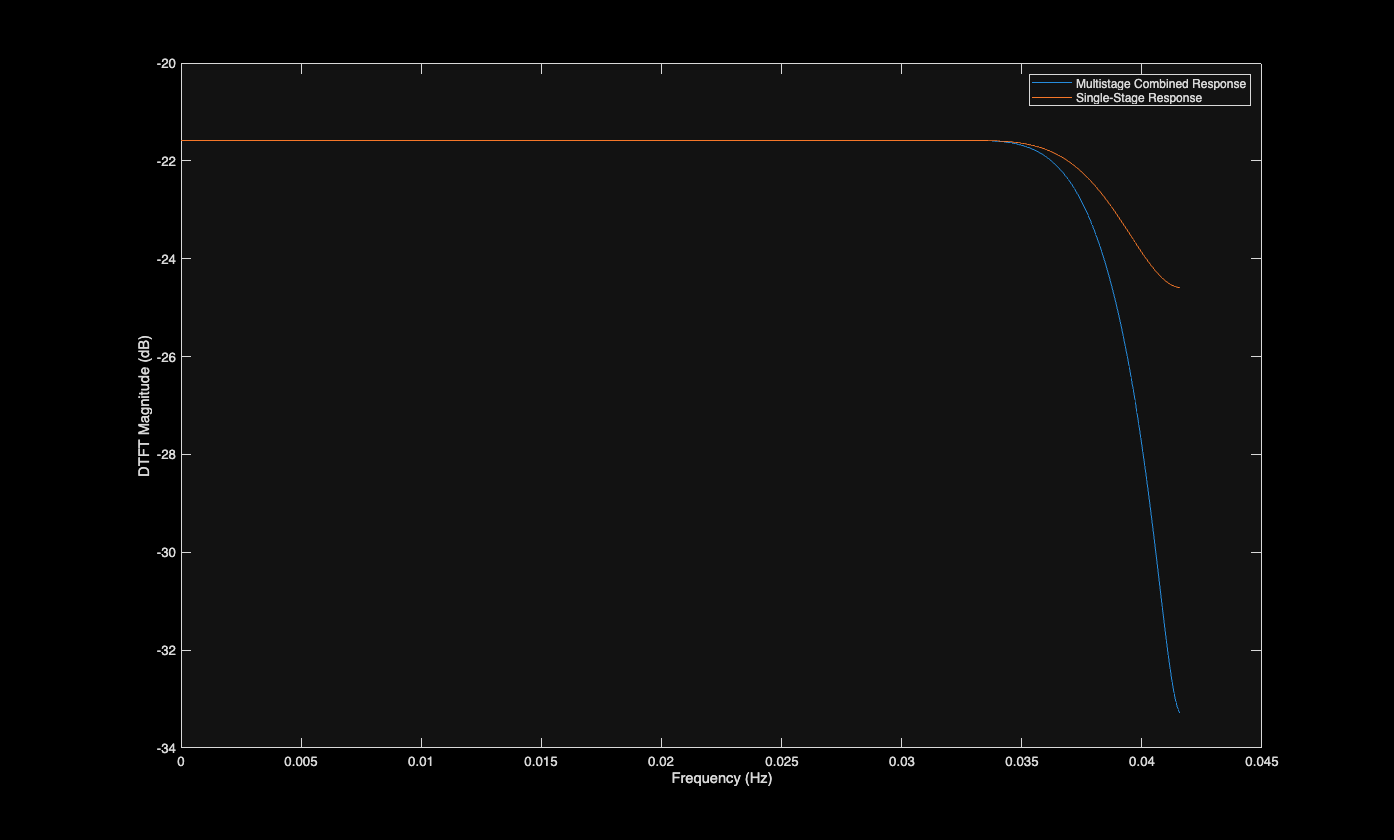### Additional Design Parameters for the designMultistageDecimator and designMultistageInterpolator Functions

You can specify filter design parameters such as transition width and stopband attenuation to the designMultistageDecimator and designMultistageInterpolator functions. Such additional parameters allow for better control of the filter characteristics. The input sample rate Fs is assumed to be 1 by default, but this value can be customized as well.

Design a filter that reduces the input rate from 48 MHz to 1 MHz, a decimation factor of 48. The following are typical specifications for a lowpass filter that reduces the bandwidth accordingly.

Fs = 48e6; TW = 100e3; Astop = 80; % Minimum stopband attenuation M = 48; % Decimation factor 

Here is a simple multistage design for these specs.

multiDecim = designMultistageDecimator(M,Fs,TW,Astop); disp(cascadeInfoSummary(multiDecim)) 
Multistage FIR Decimator, M=48 (48.0MHz to 1.0MHz) Equivalent lowpass cutoff: 1.0MHz, transition Width: 100.0kHz Number of stages: 5 Stage1: FIR Decimator, M = 2 (48.0MHz to 24.0MHz), FIR Length = 7 Stage2: FIR Decimator, M = 2 (24.0MHz to 12.0MHz), FIR Length = 7 Stage3: FIR Decimator, M = 2 (12.0MHz to 6.0MHz), FIR Length = 11 Stage4: FIR Decimator, M = 3 (6.0MHz to 2.0MHz), FIR Length = 33 Stage5: FIR Decimator, M = 2 (2.0MHz to 1.0MHz), FIR Length = 95 

This is a 5-stage decimator cascade with the factors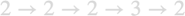whose product is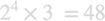as expected.

Design a similar filter with the default transition width and attenuation. The overall conversion rate is similar, but the transition width (and perhaps the ordering of the stages) can be different.

multiDecim_default = designMultistageDecimator(M,Fs); disp(cascadeInfoSummary(multiDecim_default)) 
Multistage FIR Decimator, M=48 (48.0MHz to 1.0MHz) Equivalent lowpass cutoff: 1.0MHz, transition Width: 200.0kHz Number of stages: 5 Stage1: FIR Decimator, M = 2 (48.0MHz to 24.0MHz), FIR Length = 7 Stage2: FIR Decimator, M = 2 (24.0MHz to 12.0MHz), FIR Length = 7 Stage3: FIR Decimator, M = 2 (12.0MHz to 6.0MHz), FIR Length = 11 Stage4: FIR Decimator, M = 2 (6.0MHz to 3.0MHz), FIR Length = 15 Stage5: FIR Decimator, M = 3 (3.0MHz to 1.0MHz), FIR Length = 79 

Design a single-stage decimator using the same parameters.

singleDecim = designMultistageDecimator(M,Fs,TW,Astop,'NumStages',1); disp(cascadeInfoSummary(singleDecim)) 
Multistage FIR Decimator, M=48 (48.0MHz to 1.0MHz) Equivalent lowpass cutoff: 1.0MHz, transition Width: 100.0kHz Number of stages: 1 Stage1: FIR Decimator, M = 48 (48.0MHz to 1.0MHz), FIR Length = 2411 

Compare the filter costs for the single-stage and the multistage implementations. The number of multiplications per input sample for the multistage approach is about 7, and roughly 49 for the single-stage implementation. In other words, using the multistage implementation reduces the number of multiplications by a factor of 7, which makes a significant difference. Similar differences can be observed in the number of coefficints (89 v.s. 2361), number of states (146 v.s. 2400), and additions per input sample (6 v.s. 49).

costMultiDecim = cost(multiDecim) costSingleDecim = cost(singleDecim) 
costMultiDecim = struct with fields: NumCoefficients: 89 NumStates: 146 MultiplicationsPerInputSample: 6.6042 AdditionsPerInputSample: 5.6667 costSingleDecim = struct with fields: NumCoefficients: 2361 NumStates: 2400 MultiplicationsPerInputSample: 49.1875 AdditionsPerInputSample: 49.1667 

Compare the frequency responses of the single-stage implementation and the single-stage equivalents of the two multistage designs. The gain responses of the three implementations are very similar on the passband and transition band, and have negligible differences on the stopband. In spite of the significant cost difference, the lowpass filtering in all three designs is almost the same.

hfv = fvtool(multiDecim, multiDecim_default, singleDecim); legend(hfv, 'Multistage (Custom Parameters)','Multistage (Default parameters)','Single stage')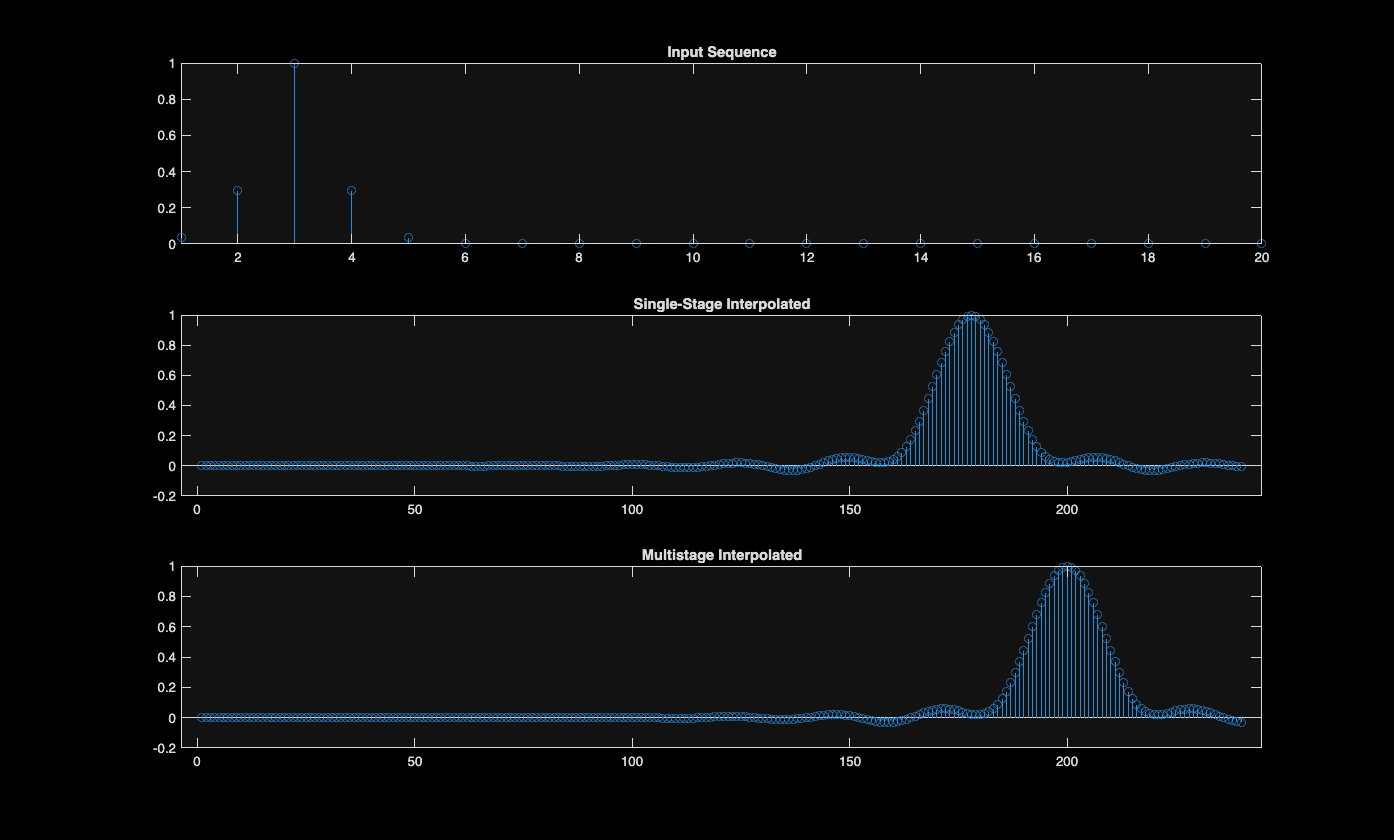The default design has a slightly larger transition width.

hfv = fvtool(multiDecim, multiDecim_default, singleDecim); legend(hfv, 'Multistage (Custom Parameters)','Multistage (Default parameters)','Single stage') xlim([0 0.8])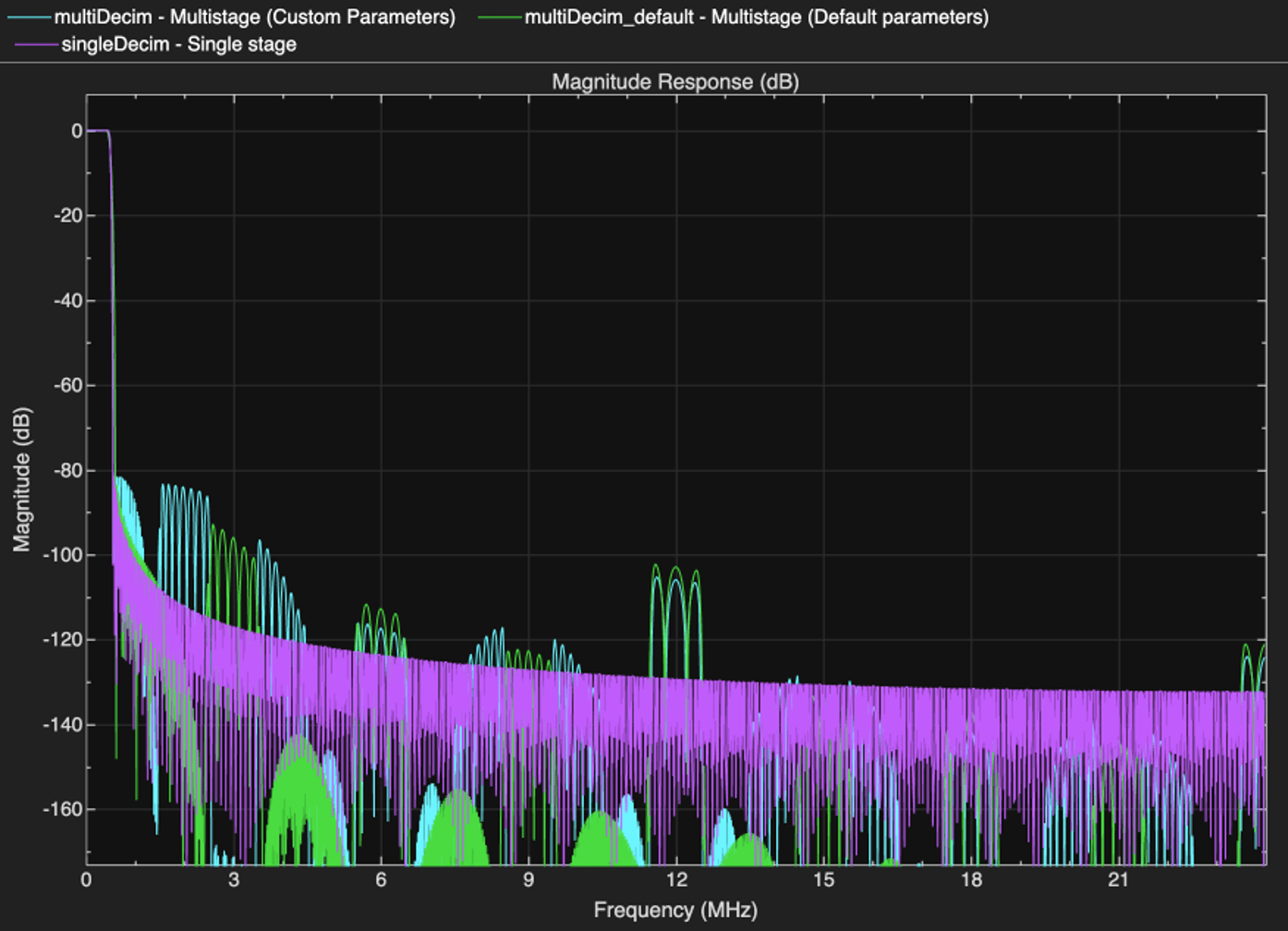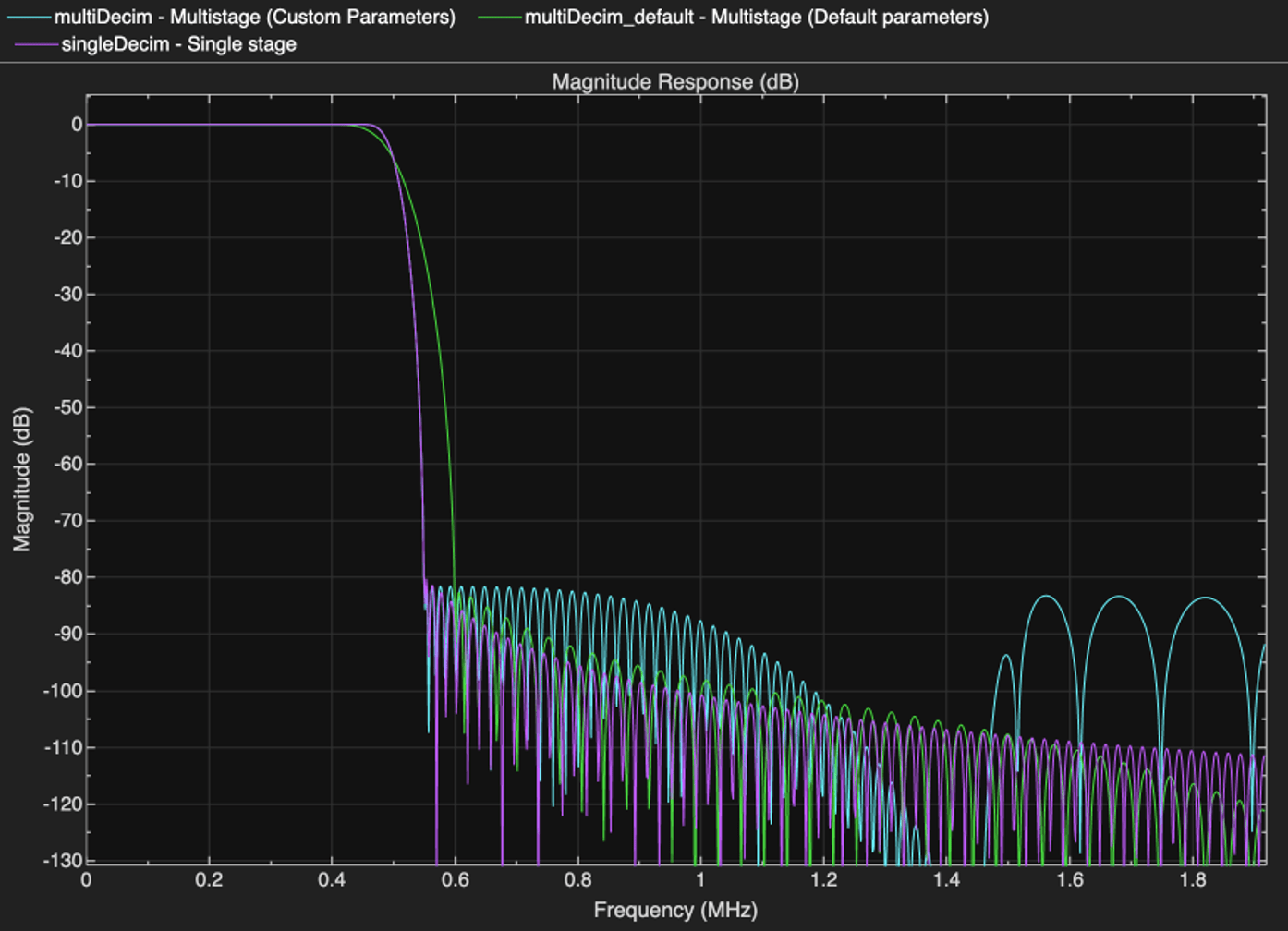### Optimial Designs for a Minimimal Total Number of Coefficients

By default, the design minimizes multiplications per input sample. It is also possible to minimize the number of coefficients. Set the property MinTotalCoeffs = true to use the latter cost.

minCoeffDecim = designMultistageDecimator(M,Fs,TW,Astop,'MinTotalCoeffs',true); disp(cascadeInfoSummary(minCoeffDecim)) cost(minCoeffDecim) 
Multistage FIR Decimator, M=48 (48.0MHz to 1.0MHz) Equivalent lowpass cutoff: 1.0MHz, transition Width: 100.0kHz Number of stages: 5 Stage1: FIR Decimator, M = 2 (48.0MHz to 24.0MHz), FIR Length = 7 Stage2: FIR Decimator, M = 3 (24.0MHz to 8.0MHz), FIR Length = 17 Stage3: FIR Decimator, M = 2 (8.0MHz to 4.0MHz), FIR Length = 11 Stage4: FIR Decimator, M = 2 (4.0MHz to 2.0MHz), FIR Length = 23 Stage5: FIR Decimator, M = 2 (2.0MHz to 1.0MHz), FIR Length = 95 ans = struct with fields: NumCoefficients: 87 NumStates: 147 MultiplicationsPerInputSample: 6.8125 AdditionsPerInputSample: 6 

Compared to multiDecim, the number of coefficients in minCoeffDecim is lower, but the number of multiplications per input sample is higher.

### Estimate vs. Design for Determining Cost

The objective function of the optimal design (either the number of coefficients or multiplications) that the designMultistageDecimator function uses depends on the FIR length of every stage. By default, this function evaluates the cost using an estimate of the FIR length rather than a true length, sometimes leading to a sub-optimal filter design.

A slower, but more precise method uses a cost based on the true FIR lengths obtained through actual designs of all filter candidates. Use the property CostMethod='design' to optimize for the accurate cost. Setting this property ensures that the design cost is indeed minimal.

trueMinCostDecim = designMultistageDecimator(M,Fs,TW,Astop, 'CostMethod','design'); disp(cascadeInfoSummary(trueMinCostDecim)) 
Multistage FIR Decimator, M=48 (48.0MHz to 1.0MHz) Equivalent lowpass cutoff: 1.0MHz, transition Width: 100.0kHz Number of stages: 5 Stage1: FIR Decimator, M = 2 (48.0MHz to 24.0MHz), FIR Length = 7 Stage2: FIR Decimator, M = 2 (24.0MHz to 12.0MHz), FIR Length = 7 Stage3: FIR Decimator, M = 3 (12.0MHz to 4.0MHz), FIR Length = 21 Stage4: FIR Decimator, M = 2 (4.0MHz to 2.0MHz), FIR Length = 23 Stage5: FIR Decimator, M = 2 (2.0MHz to 1.0MHz), FIR Length = 95 

The estimated cost performs very well in many cases (as it does in this example).

cost(trueMinCostDecim) hfv = fvtool(minCoeffDecim,trueMinCostDecim); legend(hfv, 'Optimize for Estimated FIR Lengths', 'Optimize for True FIR Lengths') 
ans = struct with fields: NumCoefficients: 87 NumStates: 146 MultiplicationsPerInputSample: 6.5625 AdditionsPerInputSample: 5.6667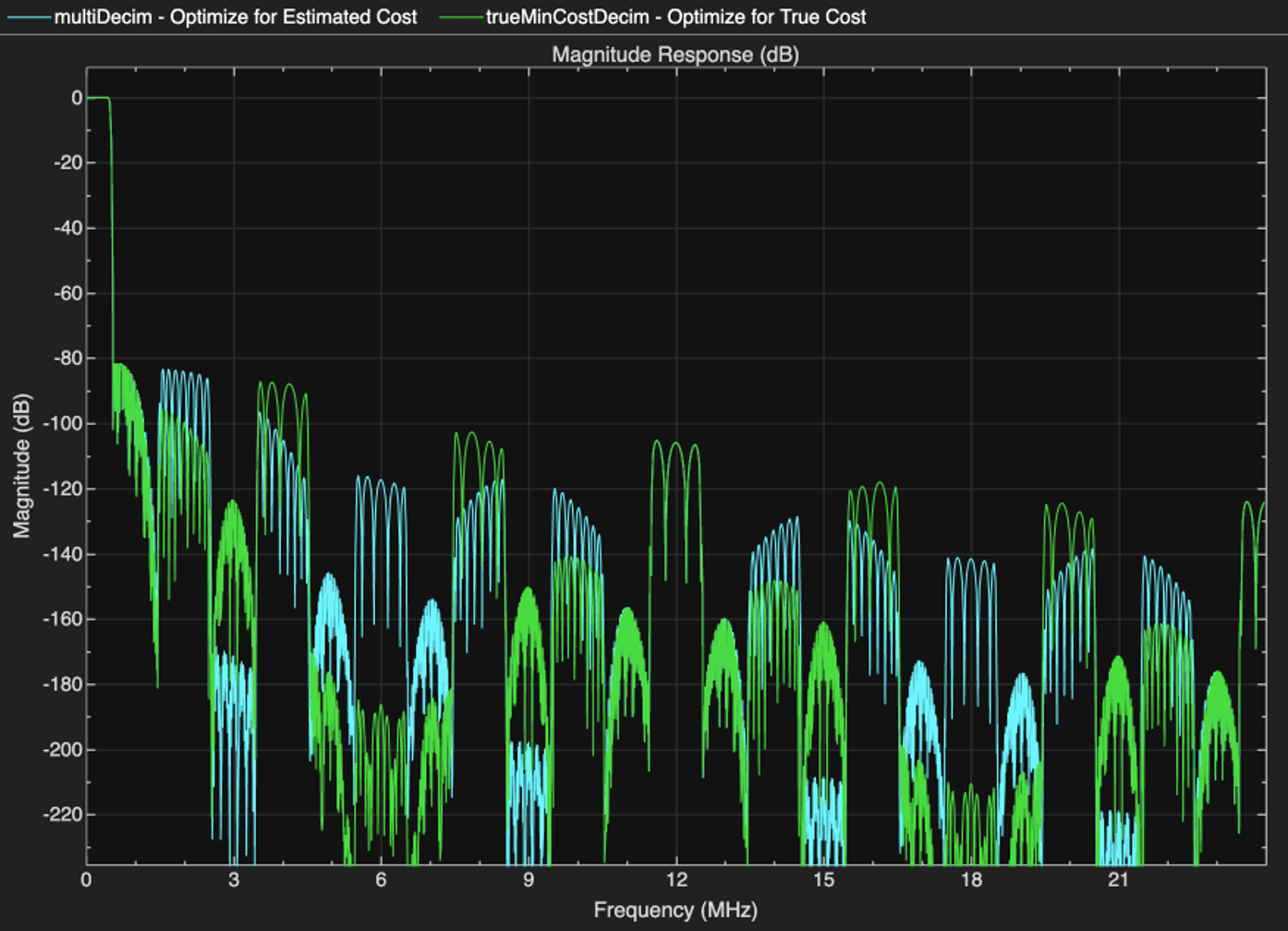### The dsp.SampleRateConverter System Object

The dsp.SampleRateConverter system object provides a convenient interface for arbitrary rate conversion, combining interpolation and decimation as needed.

src = dsp.SampleRateConverter('InputSampleRate',18,'OutputSampleRate',16,'Bandwidth',13); info(src) 
ans = 'Overall Interpolation Factor : 8 Overall Decimation Factor : 9 Number of Filters : 1 Multiplications per Input Sample: 24.333333 Number of Coefficients : 219 Filters: Filter 1: dsp.FIRRateConverter - Interpolation Factor: 8 - Decimation Factor : 9 ' 

The different stages can be extracted with the getFilters function:

firs = getFilters(src) 
firs = dsp.FilterCascade with properties: Stage1: [1x1 dsp.FIRRateConverter] CloneStages: true 

We can also specify absolute frequencies (rather than ratios). For example, the dsp.SampleRateConverter object can convert audio data sample rate from 48 kHz to 44.1 kHz.

src = dsp.SampleRateConverter('InputSampleRate',48000,'OutputSampleRate',44100); [L,M] = getRateChangeFactors(src); firs = getFilters(src); reader = dsp.AudioFileReader('audio48kHz.wav','SamplesPerFrame',4*M); x = reader(); xr = src(x); % Obtain the rate conversion FIR b = firs.Stage1.Numerator; % Calculate the resampling delay i0 = floor(length(b)/2)/L; figure; hold on; stem((1:length(x))+i0,x); stem(linspace(1,length(x),length(xr)),xr,'r'); hold off; legend('Input Audio','Resampled Audio'); xlim([150,200]) release(reader);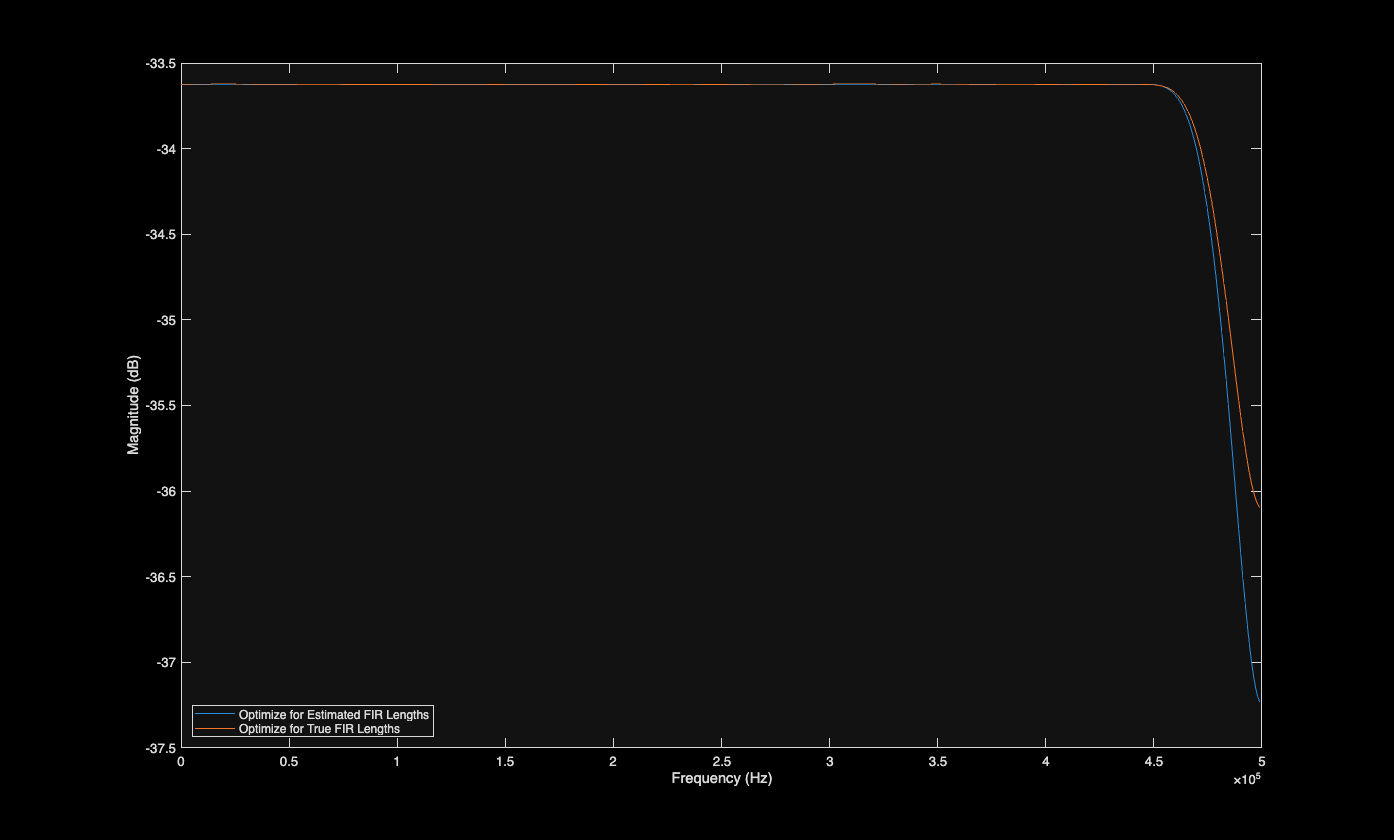### Simplification by Rate Conversion Slack

Conversion ratios like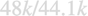(used in the previous section) requires a large upsample and downsample ratios, as even its reduced form is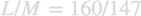. The filters required for such a conversion are fairly long, introducing a significant latency in addition to the memory and computational load.

cost(src) 
ans = struct with fields: NumCoefficients: 8587 NumStates: 58 MultiplicationsPerInputSample: 53.6688 AdditionsPerInputSample: 52.7500 

We can mitigate the costly conversion by approximating the rate conversion factor. For example,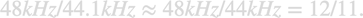The deviation of 100Hz is small, only 0.23 % of the absolute frequencies. The dsp.SampleRateConverter can automatically approximate the rate conversion factor by allowing the output frequency to be perturbed. The perturbation tolerance is specified through the 'OutputRateTolerance' property. The default tolerance is 0, meaning, no slack. In other words, slack means the deviation from the specified output rate value. Clearly, the approximated rate conversion has much smaller computational cost, and suffices for many applications, such as standard definition audio processing.

src_approx = dsp.SampleRateConverter('InputSampleRate',48000,... 'OutputSampleRate',44100,'Bandwidth',13,... 'OutputRateTolerance',0.01); [L_approx,M_approx] = getRateChangeFactors(src_approx) cost(src_approx) 
L_approx = 11 M_approx = 12 ans = struct with fields: NumCoefficients: 61 NumStates: 5 MultiplicationsPerInputSample: 5.0833 AdditionsPerInputSample: 4.1667 

 Oppenheim, A.V., and R.W. Schafer, Discrete-Time Signal Processing, Prentice-Hall, 1989.